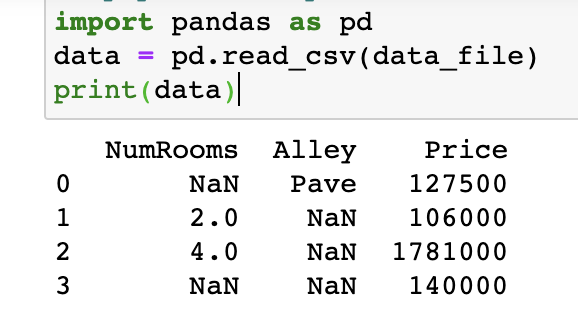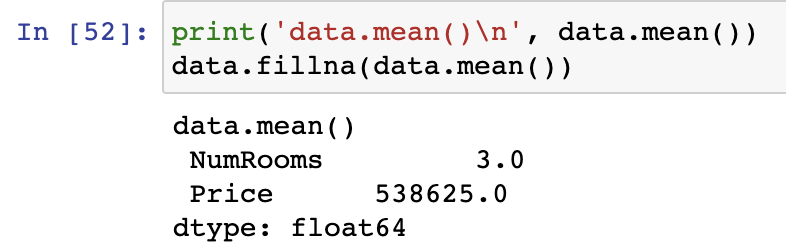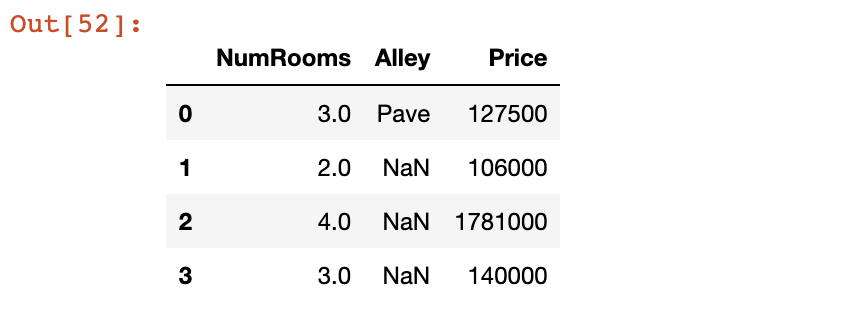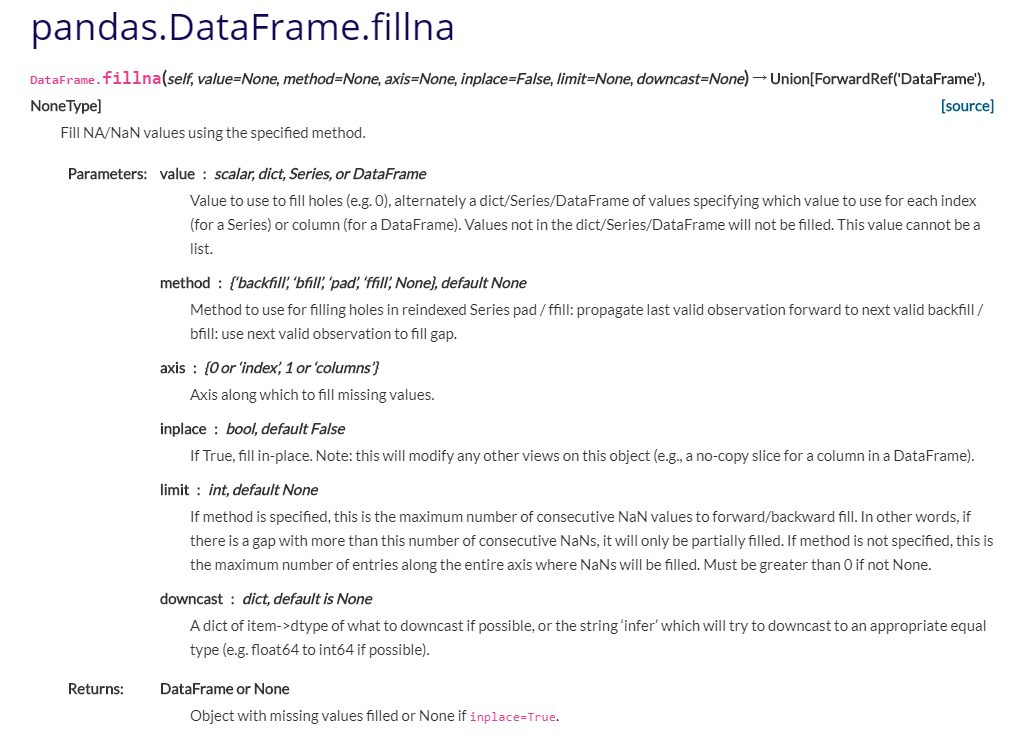• 今天小编就为大家分享一篇pandas 使用均值填充缺失值列的小技巧分享，具有很好的参考价值，希望对大家有所帮助。一起跟随小编过来看看吧
• 也就是说对于有数字的那一列的nan是可以用 那一列的平均值来替换的，但是对于字符串是不能处理的也就是说对于有数字的那一列的nan是可以用 那一列的平均值来替换的，但是对于字符串是不能处理的展开全文python
• 机器学习数据预处理之缺失值：均值填充 garbage in, garbage out. 没有高质量的数据，就没有高质量的数据挖掘结果，数据值缺失是数据分析中经常遇到的问题之一。当缺失比例很小时，可直接对缺失记录进行舍弃或...
机器学习数据预处理之缺失值：均值填充

garbage in, garbage out.
没有高质量的数据，就没有高质量的数据挖掘结果，数据值缺失是数据分析中经常遇到的问题之一。当缺失比例很小时，可直接对缺失记录进行舍弃或进行手工处理。但在实际数据中，往往缺失数据占有相当的比重。这时如果手工处理非常低效，如果舍弃缺失记录，则会丢失大量信息，使不完全观测数据与完全观测数据间产生系统差异，对这样的数据进行分析，你很可能会得出错误的结论。
平均数，统计学术语，是表示一组数据集中趋势的量数，是指在一组数据中所有数据之和再除以这组数据的个数。它是反映数据集中趋势的一项指标。解答平均数应用题的关键在于确定“总数量”以及和总数量对应的总份数。 在统计工作中，平均数（均值）和标准差是描述数据资料集中趋势和离散程度的两个最重要的测度值。
使用统计均值进行缺失值的填充；
使用fillna函数
df.fillna(df.mean())；

# 仿真数据集；
import numpy as np
import pandas as pd

# 构造数据
def dataset():
col1 = [1, 2, 3, 4, 5, 6, 7, 8, 9,10]
col2 = [3, 1, 7, np.nan, 4, 0, 5, 7, 12, np.nan]
col3 = [3, np.nan, np.nan, np.nan, 9, np.nan, 10, np.nan, 4, np.nan]
y = [10, 15, 8, 12, 17, 9, 7, 14, 16, 20]
data = {'feature1':col1, &#
展开全文数据挖掘 机器学习 深度学习 自然语言处理 人工智能
• ## 用均值填充缺失值

千次阅读 2020-10-16 21:48:44
假设数据已经通过如下代码读进来了 import pandas as pd titanic_data = pd.... 均值填充 for column in list(titanic_data.columns[titanic_data.isnull().sum() > 0]): mean_val
        假设数据已经通过如下代码读进来了
import pandas as pd


均值填充
for column in list(titanic_data.columns[titanic_data.isnull().sum() > 0]):
mean_val = titanic_data[column].mean()
titanic_data[column].fillna(mean_val, inplace=True)

pandas （sum、mean、max、min、idxmax、idxmin、cumsum、mad、std、var、diff、pct_change、corr）这些函数会默认排除NaN值         通过指定 skipna=False ，可以使其包含 Nan
展开全文• pandas中fillna()方法，能够使用指定的方法填充NA/NaN值。 1.函数详解 函数形式：fillna(value=None, method=None, axis=None, inplace=False, limit=None, downcast=None, **kwargs) 参数： value：用于填充的空值...

🥇 版权: 本文由【墨理学AI】原创、在CSDN首发、各位大佬、感谢查阅、感谢三连、感谢关注

基础参考资料

sklearn缺失值插补  sklearn官方文档  官方fillna 方法文档pandas中fillna()方法，能够使用指定的方法填充NA/NaN值。

函数详解
函数形式：fillna(value=None, method=None, axis=None, inplace=False, limit=None, downcast=None, **kwargs)
参数：
value：用于填充的空值的值。
method： {‘backfill’, ‘bfill’, ‘pad’, ‘ffill’, None}, default None。定义了填充空值的方法， pad / ffill表示用前面行/列的值，填充当前行/列的空值， backfill / bfill表示用后面行/列的值，填充当前行/列的空值。
axis：轴。0或’index’，表示按行删除；1或’columns’，表示按列删除。
inplace：是否原地替换。布尔值，默认为False。如果为True，则在原DataFrame上进行操作，返回值为None。
limit：int， default None。如果method被指定，对于连续的空值，这段连续区域，最多填充前 limit 个空值（如果存在多段连续区域，每段最多填充前 limit 个空值）。如果method未被指定， 在该axis下，最多填充前 limit 个空值（不论空值连续区间是否间断）
downcast：dict, default is None，字典中的项为，为类型向下转换规则。或者为字符串“infer”，此时会在合适的等价类型之间进行向下转换，比如float64 to int64 if possible。
返回值： DataFrame or None Object with missing values filled or None if inplace=True.

用均值进行填充:
for column in list(df.columns[df.isnull().sum() > 0]):
mean_val = df[column].mean()
df[column].fillna(mean_val, inplace=True)


用后一行的值进行填充NaN
print(df.fillna(method='backfill', axis=0, inplace=False))

我的测试代码如下：
import numpy as np
import pandas as pd

a = np.arange(100, dtype=float).reshape((10, 10))

a[0, 1] = np.nan
a[0, 3] = np.nan
a[0, 4] = np.nan
a[0, 6] = np.nan

a[3, 1] = np.nan
a[3, 3] = np.nan
a[3, 4] = np.nan
a[3, 6] = np.nan

df = pd.DataFrame(data=a)
# 重命名列名
df.columns = ['a', 'b', 'c', 'd', 'e', 'f', 'g', 'h', 'i', 'j']

print(df)
# 筛选需要填充的列
print(df.columns[df.isnull().sum() > 0])

# 用列均值进行填充NaN
for column in list(df.columns[df.isnull().sum() > 0]):
mean_val = df[column].mean()
df[column].fillna(mean_val, inplace=True)

# 用后一行的值进行填充NaN
# print(df.fillna(method='backfill', axis=0, inplace=True))

# 筛选需要填充的列  发现没有这样的列了
print(df.columns[df.isnull().sum() > 0])

print(df)展开全文pandas
• 使用transform： >>> df name value 0 A 1 1 A NaN 2 B NaN 3 B 2 4 B 3 5 B 1 6 C 3 7 C NaN 8 C 3 >... df["value"] = df.groupby("name").transform(lambda x: x.fillna(x.mean()))数据处理 pandas
• pd.DataFrame中通常含有许多特征，有时候需要对每个含有缺失值的列，都用均值进行填充，代码实现可以这样： for column in list(df.columns[df.isnull().sum() > 0]): mean_val = df[column].mean() df[column]....
• 今天小编就为大家分享一篇Python Pandas实现数据分组求平均值并填充nan的示例，具有很好的参考价值，希望对大家有所帮助。一起跟随小编过来看看吧
• 利用各行/各列的均值填充空值 参考： 1：python—创建字典的方式 2：pandas.DataFrame.fillna¶ 利用各行/各列的均值填充空值   以列为例，简单来说，填充时指定各列填充的值为各列的均值就好了。对行的操作，...
• 在奇异值阈值法的基础上,针对循环矩阵的特殊结构分别对一般低秩复循环矩阵和特殊低秩实循环矩阵作保结构的均值算法.首先给出构造低秩循环矩阵的方法;其次,给出了修正的保结构算法;最后通过数值实验验证结果.
• 均值填充（Mean/Mode Completer） 将信息表中的属性分为数值属性和非数值属性来分别进行处理。如果空值是数值型的，就根据该属性在其他所有对象的取值的平均值来填充该缺失的属性值；如果空值是非数值型的，就根据...
• 下面小编就为大家分享一篇pandas对指定列进行填充的方法，具有很好的参考价值，希望对大家有所帮助。一起跟随小编过来看看吧
• 将其Nan全部填充为0，这时再打印的话会发现根本未填充，这是因为没有加上参数inplace参数。 一定要将inplace = True加入参数，这样才能让源数据发生改变并保存。 >>> df.fillna(0, inplace = True) >>> print(df) #...
• = 0: # 说明当前列有num # 选中当前列不为nan的元素组成的数组，求出他们的均值，将均值赋值给该列nan的元素 temp_not_nan_col = temp_col[temp_col == temp_col] # temp_col==temp_col返回一个数组，里面为true和...numpy python
• 比如，用fillna()填充 # 用中位数填充 X_missing.loc[:,"age"] = X_missing.loc[:,"age"].fillna(X_missing.loc[:,"age"].median()) 或者更一般的 # 均值填充 data['col'] = data['col'].fillna(data['col'].means...
• df = df.fillna(df.interpolate()) fillna() 括号里面还可以填其他参数 参数 解释 ...用后一个数据填充 df = df.fillna(method='bfill') pad 用前一个数据填充 df = df.fillna(method='pad')pandas
• 找出文档中的空值，并用前值填充空值，分为大跳（中间有连续空值），和小跳，中间只有一个空值
• import numpy as np # # 用每一列的平均值填充 ，np.mean() t=np.arange(24,dtype=float).reshape(4,6) t[3,:] = np.nan #初始有空值的数组 ... lie[np.isnan(lie)]=tc #用均值填充空值 t#打印填充完之后的tpython
• 填充均值 //连续值填充均值 def ContinuousMissValueProcessingMean(df:DataFrame,douCols:Array[String]):DataFrame= { println("----连续值填充均值----开始-----") val meanDF = df.select((douCols)...spark dataframe
• 文章目录概述缺失值的常用处理方式sklearn中缺失值填充模块缺失值填充示例准备工作0填充均值填充众数填充中位数填充随机森林填充总结 概述 机器学习和数据挖掘中所使用的数据，永远不可能是完美的。很多特征，对于...python 数据分析 机器学习 pandas 经验分享
• ## 数据处理之缺失值填充

千次阅读 多人点赞 2020-04-18 18:42:44
（4.1）方法一（.fillna()） import numpy as np import pandas as pd data = pd.read_csv('1.csv') data['C1'] = data['C1'].fillna(data['C1'].mean()) # 均值填充：.mean()--->.median()--->.mode() data[50:60]......